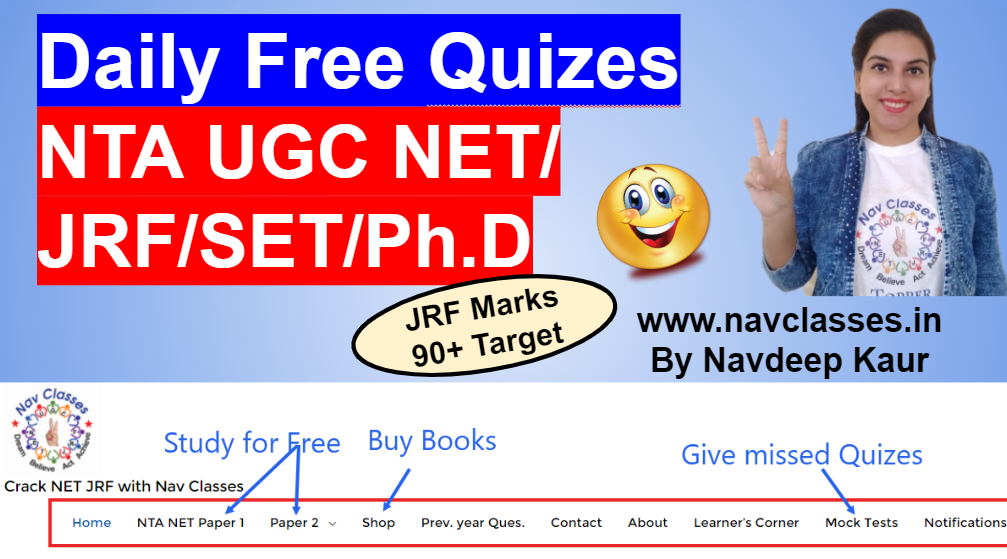# Logical Reasoning for NTA UGC NET JRF Paper 1

1. Answer the questions based on the number sequence below
543 589 657 758 732 685
If the first and last digits of each number are interchanged what would be the second largest number?
a) 756
b) 857
c) 985
d) 874

Explanation: The required sequence would be: 345 985 756 857 237 586

2. Answer the questions based on the number sequence below
543 589 657 758 732 685

Which number in the sequence has the highest sum of its digits?
a) 758
b) 732
c) 685
d) 589

Explanation: 543= 5+4+3= 12 589= 5+8+9= 22
657= 6+5+7= 18
758= 7+5+8= 20
732= 7+3+2= 12
685= 6+8+5= 19.

3. Statement: Some leaders are as corrupts as Mahesh.
Conclusion:
a) Mahesh is corrupt.
b) Most of the leaders are corrupt.
c) Mahesh is not corrupt.

Explanation: The statement clearly tells Mahesh is corrupt but nothing can be said about the leaders.

4. In a cinema hall, 8 friends, H, I, J, K, L, M, N, O are sitting in a straight line according to the following rules:
• J is the neighbour of K.
• H is just next to the left of L.
• I is in one of the two seats in the middle.
• M is the only one sitting between I and L.
If N is not sitting at the extreme end, then who occupies the extreme right seat?
a) H
b) J
c) K
d) None of these

Explanation: The possible Answers are: H N/O L M I O/N J/K K/J
Thus, Either J or K occupies extreme right seat.

5. If OUGHT is coded as ABCDE in a certain language. How can TOUGH be coded in the same language?

a) ABCDE
b) BCDEA
c) CDEAB
d) EABCD

Explanation: Here, the code for O = A, U = B, G = C, H = D and T = E. Then, the code for TOUGH is EABCD.

6. If BITER is coded as KLMNO in a certain language. How can TRIBE be coded in the same language?

a) MOLNK
b) MOLKN
c) MOKLN
d) MOKNL

Explanation: Explanation: Here, the code for B = K, I = L, T = M, E = N and R = O. Then, the code for TRIBE is MOLKN.

7. Pointing to a man in the Photograph Kabir said, ‘His son’s mother is the only daughter in law of my mother’. Who is the person in photograph to Kabir?

a) Brother
b) Uncle
c) Father
d) None of these

Explanation: The person is Kabir himself.

8. Rahul and Govind start a race, and together they run for 50m. Rahul turns right and runs 60m. While Govind turns left and runs 40m. Then Rahul turns left and runs 50m. While Govind turns right and runs 50m. How far are both now from the each other?

a) 60m
b) 100m
c) 20m
d) 150m

Explanation: Rahul and Govind will finish on a straight line and the distance between them will be = 40 + 60 = 100m.

9. How many such letters are there in the word “ACCELERATION” each of which is as far away from the beginning of the word as it is from the beginning of the English Alphabet?

a) None
b) One
c) Two
d) Three

Explanation: Clearly, C is the third letter in the word “ACCELERATION” as well as in the English Alphabet. Therefore, there is only one such letter.

10. In a certain code, ABET is written as 3-5-11-41, how will CUBE be written in same code?

a) 3-41-5-11
b) 9-35-5-11
c) 1-17-5-11
d) 7-43-5-11

Explanation: Coding pattern is (2n + 1) where n = position of alphabet in alphabetic order.

1.